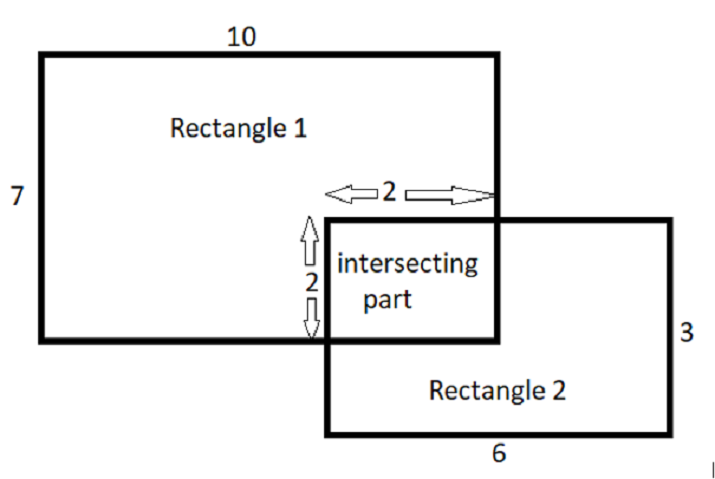# How to find total area when two rectangles overlap each other in Java?

A Rectangle is a four sided-polygon, with opposite side equal and having all the internal angles equal to 90 degrees. In this article we will check how to find total area when two rectangles overlap each other.

We will be given with two rectangle having length and breadth. Also we will we given with intersecting length and width of the two rectangles as shown in the figure −To find the total area of a rectangle we basically add areas of two rectangles. This includes the intersecting part twice, so we subtract the area of intersecting part.

Total Area = (Area of 1st rectangle + Area of 2nd rectangle) - Area of Intersecting part


Let’s start!

## To show you some instances

### Instance-1

Given the length and breadth of rectangle are −

Rectangle 1: length = 16, breadth = 25,
Rectangle 2: length = 12, breadth = 13,
Rectangle 3: length = 9, breadth = 3,

• After putting it in the formula, the result will be −

• The resulting area is: 529

### Instance-2

Given the length and breadth of rectangle are −

Rectangle 1: length = 10, breadth = 7,
Rectangle 2: length = 6, breadth = 3,
Rectangle 3: length = 2, breadth = 2,

• After putting it in the formula, the result will be −

• The resulting area is: 84

### Algorithm

• Step-1 − Declare and initialize the variables.

• Step-2 − Putting the values in formula.

• Step-3 − Print the result.

## Multiple Approaches

We have provided the solution in different approaches.

• By Using Static Inputs

• By Using User Defined Method

Let’s see the program along with its output one by one.

## Approach-1: By Using Static Input

In this approach, length and breadth of rectangles will be assigned. Then as per the algorithm we will find total area when two rectangles overlap each other.

### Example

public class Main {

//main method
public static void main (String[] args){

//Declaring variables
int l1 = 16, b1 = 25;
int l2 = 12, b2 = 13;
int l3 = 9, b3 = 3;

//applying logic
int A1 = l1 * b1;
int A2 = l2 * b2;
int A3 = l3 * b3;
int A4 = A1 + A2 - A3;

//printing the result
System.out.println("The resulting area is: " + A4);
}
}


### Output

The resulting area is: 529


## Approach-2: By Using Stream

In this approach, length and breadth of rectangles will be assigned. Then call a user defined method by passing the given values and as per the algorithm we will find total area when two rectangles overlap each other.

### Example

public class Main {

//main method
public static void main (String[] args){

//Declaring variables
int l1 = 10, b1 = 7;
int l2 = 6, b2 = 3;
int l3 = 2, b3 = 2;

//calling user defined method
func(l1, l2, l3, b1, b2, b3);
}

//user defined method
static void func(int l1, int l2, int l3, int b1, int b2, int b3){

//applying logic
int A1 = l1 * b1;
int A2 = l2 * b2;
int A3 = l3 * b3;
int A4 = A1 + A2 - A3;

//printing the result
System.out.println("The resulting area is: " + A4) ;
}
}


### Output

The resulting area is: 84


In this article, we explored different approaches to find total area when two rectangles overlap each other by using Java programming language.

Updated on: 04-May-2023

106 Views